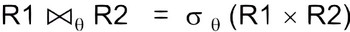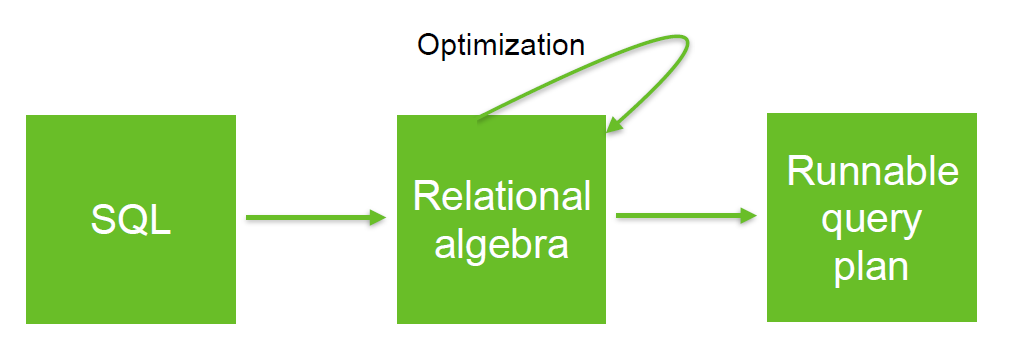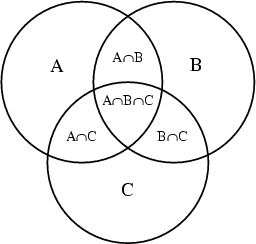# Relational Operator - Cross Product

The set cartesian product applied to a set of row (ie a tables) create all pairs of tuples (row).

A cross-join (also called Cartesian join) occurs when a request does not have a join condition between two tables. We say that the inner table is not driven from (not dependent on) the outer table.

That creates a cartesian product of the tables involved in the join. The size of a cartesian product is the number of rows in the first table multiplied by the number of rows in the second table.

In other word, if the inner table's access path is independent of the outer table, then the same rows are retrieved for every iteration of the outer loop.

The cartesian product in named for Rene Descartes.

## Symbol

A cross (times) $$\LARGE R1 \times R2$$

where:

• the x character is:

## Size

The size of this cross-join is then the size of the first table (R1) multiplied by the size of the second table (R2).

## Example

### Sql

Discover MoreLogical Data Modeling - Duplicate

A duplicate happens when in a set two elements have an equivalence relationship that returns true. In the set {1,1,2,3}, 1 is a duplicate The set {1,2,3} has no duplicate relational operators...Relational Algebra - Theta-join

A theta-join is a difficult/complex join where the condition is not a equality. Example: Band join or range join. A theta is a join that links tables based on a relationship other than the equality...Relational Operator - Equi-joins

An Equi-join is a join where the condition (predicate) is an equality. theta join An equi-join links two relations (tables, ...) on common values in a column in relation 1 with a column in relation...Relational Operator - Join

join is a relational operator that pair tuples (row) of two relations (table, ...) according to a condition (predicate). where: c is a condition (ie predicate) See Type Symbol Unicode...Relational Operator - Rename

Rename or (renaming) is a relational operator that rename: a relation or an attribute of the relation rename the attribute a from R to b as Rename the 4 attributes {A, B, A, C} resulting...SQL - Cross Join / Cartesian Product / Cross Product

in SQL The data below is used in the explicit and implicit example table1 (inner set) table2 (outer set) Column ID Column ID Column ID_2 A A B B A D C C And we want: We have...Set Theory - Cross Product (Cartesian product)

The cartesian product is a set operator between two set A and B that produces a set of all pairs (a, b) where: (ie a in A) and (ie b in B) See See